#cfa #economics #has-images

Mathematically, the inverse relationship of the Law of Demand may be expressed as a causal relation: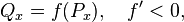where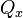is the quantity demanded of good x,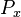is the price of the good,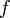is the demand function, and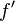is its derivative.

Here,is the causal factor (independent variable) andIs the dependent variable

If you want to change selection, open document below and click on "Move attachment"

Law of demand - Wikipedia, the free encyclopedia
ction 3 Exceptions to the law of demand 3.1 Giffen goods 3.2 Expectation of change in the price of commodity 3.3 Basic or necessary goods 4 The law of demand and change in demand 5 See also 6 References Mathematical expression <span>Mathematically, the inverse relationship may be expressed as a causal relation: where is the quantity demanded of good x, is the price of the good, is the demand function, and is its derivative. Here, is the causal factor (independent variable) and Is the dependent variable. Graphical depiction A demand curve is a graphical depiction that abides by the law of demand. It shows how the quantity demanded of some product during a specified period of